### Difference Between Plane and Geodetic Surveying

Plane surveying and Geodetic Surveying are two major classifications that follows two different surveying strategies in making measurement of points or distances on, below or above the earth surface.Difference Between Plane and Geodetic Surveying

In more easy terms, plane surveying is the surveying conducted by assuming the earth surface is flat. Here the spheroidal shape or curvature of earth (Oblate) surface is ignored, while geodetic survey involves the survey of extensive areas that needs to take into consideration the curvature of the earth, shape of earth and size of earth.

 Sl.No: Plane Surveying Geodetic Surveying 1 Plane surveying is a surveying performed by considering the mean surface of the earth as plane. Geodetic surveying is a surveying in which the mean surface of the earth is considered as spherical. 2 The plumb bob lines passing through the points are parallel to each other in plane surveying.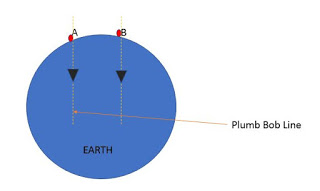Plumb bob lines passing through the points intercept at the centre of the earth in geodetic surveying.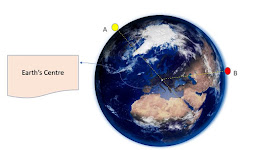3 The lines that are formed by joining two points on earth during plane surveying is considered as straight lines.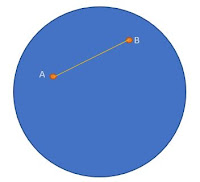The lines that are formed by joining two points on earth surface during geodetic surveying is curved lines or arc lines.4 Hence, the triangles formed in plane surveying are plane triangles.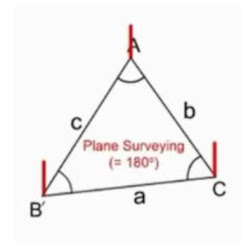The triangles formed in geodetic surveying are spherical triangles.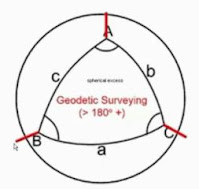5 The triangles formed are solved using the knowledge of plane trigonometry. In geodetic surveying, the triangles are solved using the knowledge of spherical trigonometry. 6 Plane surveying is performed by using normal survey and measuring instruments like chain, theodolite, measuring tape etc. Geodetic surveying make use of more sophisticated and precise instruments and modern technology 7 The measurement accuracy is low compared to geodetic survey. Geodetic surveying possess higher degree of accuracy. 8 Plane surveying is employed for areas less than 260km2. Geodetic surveying is employed for areas greater than 260km2. 9 Plane surveying is used for general civil engineering survey projects. These are used to perform less precision works and locate points with respect to control stations that are obtained through geodetic surveying. Geodetic survey is used to locate precise positions of distant areas. Most of the points determined and located forms the control stations. 10 Plane surveying is performed by individual organization or firms for performing a construction project. Geodetic surveying is performed by governments and state departments. 11 Plane Surveying is easy and economical. Geodetic surveying is complex and hence costly type of surveying.

1.